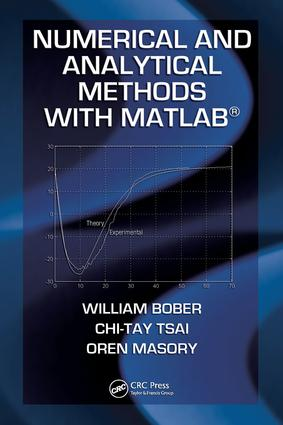# Numerical and Analytical Methods with MATLAB

## 1st Edition

CRC Press

472 pages

Hardback: 9781420093568
pub: 2009-08-11
\$150.00
x
eBook (VitalSource) : 9780429150937
pub: 2009-08-11
from \$75.00

FREE Standard Shipping!

### Description

Numerical and Analytical Methods with MATLAB presents extensive coverage of the MATLAB programming language for engineers. It demonstrates how the built-in functions of MATLAB can be used to solve systems of linear equations, ODEs, roots of transcendental equations, statistical problems, optimization problems, control systems problem

Numerical Modeling for Engineering. MATLAB Fundamentals. Matrices. Roots of Algebraic and Transcendental Equations. Numerical Integration. Numerical Integration of Ordinary Differential Equations. Simulink. Curve Fitting. Optimization. Partial Differential Equations. Iteration Method. Laplace Transforms. An Introduction to the Finite Element Method. Control Systems. Appendices. Index.

William Bober, Chi-Tay Tsai, and Oren Masory are all professors in the Department of Mechanical Engineering at Florida Atlantic University.